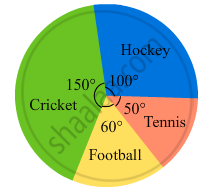Share

# The Pie Chart (As Shown in the Figure 25.23) Represents the Amount Spent on Different Sports by a Sports Club in a Year. If the Total Money Spent by the Club on Sports is Rs 1,08 - Mathematics

Course
ConceptIntroduction of Circle Graph Or Pie Chart

#### Question

The pie chart (as shown in the figure 25.23) represents the amount spent on different sports by a sports club in a year. If the total money spent by the club on sports is Rs 1,08,000, find the amount spent on each sport.#### Solution

$\text{ Amount spent on cricket }= \frac{\text{ Central angle of the corresponding sector }\times \text{ Total Money spent }}{360^\circ}$
$= \frac{150^\circ \times 108000}{360^\circ} = Rs 45, 000$
$\text{ Amount spent on hockey }= \frac{\text{ Central angle of the corresponding sector }\times\text{ Total Money spent }}{360^\circ}$
$= \frac{100^\circ \times 108000}{360^\circ} = Rs 30, 000$
$\text{ Amount spent on football }= \frac{\text{ Central angle of the corresponding sector }\times\text{ Total Money spent }}{360^\circ}$
$= \frac{60^\circ \times 108000}{360^\circ} = Rs 18, 000$
$\text{ Amount spent on tennis }= \frac{\text{ Central angle of the corresponding sector }\times\text{ Total Money spent }}{360^\circ}$
$= \frac{50^\circ \times 108000}{360^\circ} = Rs 15, 000$

Is there an error in this question or solution?

#### APPEARS IN

RD Sharma Solution for Mathematics for Class 8 by R D Sharma (2019-2020 Session) (2017 to Current)
Chapter 25: Data Handling-III (Pictorial Representation of Data as Pie Charts or Circle Graphs)
Ex. 25.2 | Q: 7 | Page no. 23

#### Video TutorialsVIEW ALL 

Solution The Pie Chart (As Shown in the Figure 25.23) Represents the Amount Spent on Different Sports by a Sports Club in a Year. If the Total Money Spent by the Club on Sports is Rs 1,08 Concept: Introduction of Circle Graph Or Pie Chart.
S